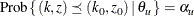### Analysis after a Sequential Test

At the end of a trial, the hypothesis is either rejected or accepted. But the p-value, median, and confidence limits depend on the ordering the sample space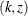, where k is the stage number and z is the standardized Z statistic.

Following the notations used in Jennison and Turnbull (2000, pp. 179–180),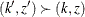if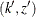has a higher order or more extreme than. Then for a given ordering, the p-value, median, and confidence limits associated with the observed statisticscan be derived.

#### p-value

With the observed pair of statistics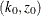when the trial is stopped, a one-sided upper p-value is computed as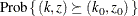A one-sided lower p-value is computed as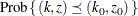A two-sided p-value is twice the smaller of the lower and upper p-values.

#### Median Unbiased Estimate

With the observed pair, a median unbiased estimate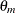is computed from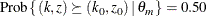#### Confidence Limits

With the observed pair, a lower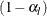confidence limit for,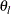, is computed from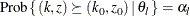Similarly, an upper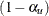confidence limit for,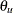, is computed from